# Electronics and Communication Engineering - Exam Questions Papers

16.

1. Hall coefficient RH is directly proportional to the charge density
2. RH depends upon type of the semiconductor material (n - type or p - type)
3. Hall co-efficient RH is directly proportional to hall voltage VH
From the above statements which are the false statements?

 A. I only B. II only C. III only D. all of the above

Explanation: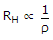where r is charge density

and RH = + ve, then the material is p - type

= - ve, then the material is n - type

RH ∝ VH where VH is Hall voltage.

17.

The equivalent form of the logical expression (ABC + ABC + ABC + ABC + ABC) is:

 A. C + (A ⊕ B) B. (A + B + C)(A + B + C) C. (A + B + C)(A + B + C)(A + B + C) D. (A + B + C)(A + B + C)(A + B + C)

Explanation:

K-map for the expression: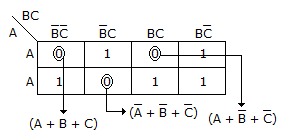18.

In a series operated thyristor configuration, each thyristor should support 100 V. However, the three thyristors only support voltage of 100 V, 90 V and 85 V respectively. Hence, the string efficiency in the case is :

 A. 98.45% B. 45.84% C. 91.67% D. none of these

Explanation:

String efficiency =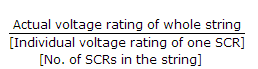=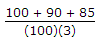= 0.9167 = 91.67%.

19.

A uniform plane wave in the free space is normally incident on an infinitely thick dielectric slab (dielectric constant εr = 9). The magnitude of the reflection coefficient is

 A. 0 B. 0.3 C. 0.5 D. 0.8

Explanation: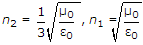Thus reflection coefficient =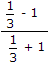= - 0.5

Thus magnitude is 0.5 .

20.

A speech signal, band limited to 4kHz and peak voltage varying between + 5V and - 5V is sampled at the Nyquist rate. Each sample is quantized and represented by 8 bits. If the bits 0 and 1 are transmitted using bipolar pulses, the minimum bandwidth required for distortion free transmission is

 A. 64 kHz B. 32 kHz C. 8 kHz D. 4 kHz

Explanation:

According to Nyquist Criteria, minimum sampling frequency = 2 x 4 k = 8 kHz

But each cycle can accommodate two bits

Thus minimum frequency = 4 kHz.

#### Current Affairs 2022

Interview Questions and Answers